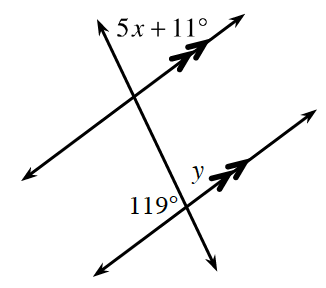### Home > INT2 > Chapter 1 > Lesson 1.3.4 > Problem1-113

1-113.

Examine the angle pair relationships in the diagram at right. Then write and solve equations for $x$ and $y$, if possible. Justify your work using angle relationships.

$∠119^\circ$ and $∠y$ are supplementary angles, and $∠y$ and $∠5x+11^\circ$ are corresponding angles.

See the homework help for problem 2-55 in Lesson 2.1.5 if you need further help solving for $x$ and $y$.